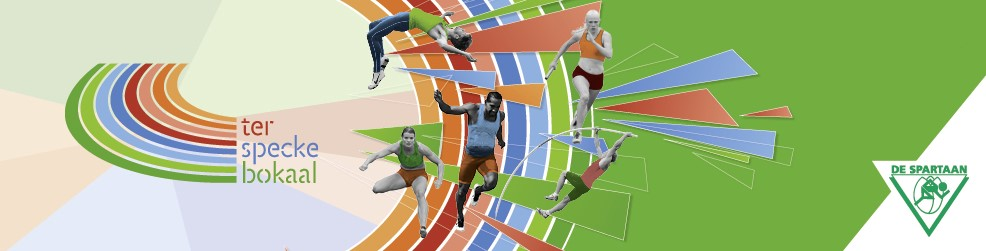# Results

The results of the 37th Ter Specke Bokaal can be found here. (2019)

The results of the 36th Ter Specke Bokaal can be found here. (2018)

The results of the 35th Ter Specke Bokaal can be found here. (2017)

The results of the 34th Ter Specke Bokaal can be found here. (2016)

The results of the 33rd Ter Specke Bokaal can be found here. (2015)

The results of the 32nd Ter Specke Bokaal can be found here. (2014)

The results of the 31st Ter Specke Bokaal can be found here. (2013)

The results of the 30th Ter Specke Bokaal can be found here. (2012)

The results of the 29th Ter Specek Bokaal can be found here. (2011)

The results of the 28th Ter Specke Bokaal can be found here. (2010)

The results of the 27th Ter Specke Bokaal can be found here. (2009)

The results of the 26th Ter Specke Bokaal can be found here. (2008)

The results of the 25th Ter Specke Bokaal can be found here. (2007)
Terug naar boven